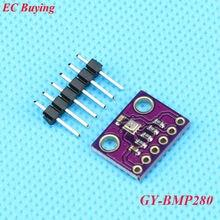# Beaglebone and BMP280 sensor example in C

In this article we look at an BMP280 connected to a Beaglebone first of all lets look at the sensor.

BMP280 is an absolute barometric pressure sensor especially designed for mobile applications. The sensor module is housed in an extremely compact package. Its small dimensions and its low power consumption allow for the implementation in battery powered devices such as mobile phones, GPS modules or watches.

As its predecessor BMP180, BMP280 is based on Bosch’s proven Piezo-resistive pressure sensor technology featuring high accuracy and linearity as well as long term stability and high EMC robustness. Numerous device operation options offer highest flexibility to optimize the device regarding power consumption, resolution and filter performance. A tested set of default settings for example use case is provided to the developer in order to make design-in as easy as possible.Applications

– Indoor navigation (floor detection, elevator detection)

– Outdoor navigation, leisure and sports applications

– Weather forecast

– Health care applications (e.g. spirometry)

– Vertical velocity indication (e.g. rise/sink speed)

Parameter Technical data
Operation range (full accuracy) Pressure: 300…1100 hPa
Temperature: -40…85°C
Absolute accuracy
(Temp. @ 0…+65°C)
~ ±1 hPa
Relative accuracy
p = 700…900hPa
(Temp. @ +25…+40°C)
± 0.12 hPa (typical)
equivalent to ±1 m
Average current consumption (1 Hz data refresh rate) 2.74 μA, typical
(ultra-low power mode)
Average current consumption in sleep mode 0.1 μA
Average measurement time 5.5 msec
(ultra-low power preset)
Supply voltage VDDIO 1.2 … 3.6 V
Supply voltage VDD 1.71 … 3.6 V
Resolution of data Pressure: 0.01 hPa ( < 10 cm)
Temperature: 0.01° C
Temperature coefficient offset
(+25°…+40°C @900hPa)
± 0.12 hPa (typical)
equivalent to ±1 m
Interface I²C and SPI

### Code Example

This is a controleverything example – they have code examples for various platforms. This is the C example from https://github.com/ControlEverythingCommunity/BMP280

[codesyntax lang=”cpp”]

```#include <stdio.h>
#include <stdlib.h>
#include <linux/i2c-dev.h>
#include <sys/ioctl.h>
#include <fcntl.h>
#include <math.h>

void main()
{
// Create I2C bus
int file;
char *bus = "/dev/i2c-2";
if((file = open(bus, O_RDWR)) < 0)
{
printf("Failed to open the bus. \n");
exit(1);
}
// Get I2C device, BMP280 I2C address is 0x76(108)
ioctl(file, I2C_SLAVE, 0x76);

char reg = {0x88};
write(file, reg, 1);
char data = {0};
{
printf("Error : Input/output Error \n");
exit(1);
}
// Convert the data
// temp coefficents
int dig_T1 = data * 256 + data;
int dig_T2 = data * 256 + data;
if(dig_T2 > 32767)
{
dig_T2 -= 65536;
}
int dig_T3 = data * 256 + data;
if(dig_T3 > 32767)
{
dig_T3 -= 65536;
}
// pressure coefficents
int dig_P1 = data * 256 + data;
int dig_P2  = data * 256 + data;
if(dig_P2 > 32767)
{
dig_P2 -= 65536;
}
int dig_P3 = data* 256 + data;
if(dig_P3 > 32767)
{
dig_P3 -= 65536;
}
int dig_P4 = data* 256 + data;
if(dig_P4 > 32767)
{
dig_P4 -= 65536;
}
int dig_P5 = data* 256 + data;
if(dig_P5 > 32767)
{
dig_P5 -= 65536;
}
int dig_P6 = data* 256 + data;
if(dig_P6 > 32767)
{
dig_P6 -= 65536;
}
int dig_P7 = data* 256 + data;
if(dig_P7 > 32767)
{
dig_P7 -= 65536;
}
int dig_P8 = data* 256 + data;
if(dig_P8 > 32767)
{
dig_P8 -= 65536;
}
int dig_P9 = data* 256 + data;
if(dig_P9 > 32767)
{
dig_P9 -= 65536;
}

// Select control measurement register(0xF4)
// Normal mode, temp and pressure over sampling rate = 1(0x27)
char config = {0};
config = 0xF4;
config = 0x27;
write(file, config, 2);

// Select config register(0xF5)
// Stand_by time = 1000 ms(0xA0)
config = 0xF5;
config = 0xA0;
write(file, config, 2);
sleep(1);

// Read 8 bytes of data from register(0xF7)
// pressure msb1, pressure msb, pressure lsb, temp msb1, temp msb, temp lsb, humidity lsb, humidity msb
reg = 0xF7;
write(file, reg, 1);
{
printf("Error : Input/output Error \n");
exit(1);
}

// Convert pressure and temperature data to 19-bits
long adc_p = (((long)data * 65536) + ((long)data * 256) + (long)(data & 0xF0)) / 16;
long adc_t = (((long)data * 65536) + ((long)data * 256) + (long)(data & 0xF0)) / 16;

// Temperature offset calculations
double var1 = (((double)adc_t) / 16384.0 - ((double)dig_T1) / 1024.0) * ((double)dig_T2);
double var2 = ((((double)adc_t) / 131072.0 - ((double)dig_T1) / 8192.0) *(((double)adc_t)/131072.0 - ((double)dig_T1)/8192.0)) * ((double)dig_T3);
double t_fine = (long)(var1 + var2);
double cTemp = (var1 + var2) / 5120.0;
double fTemp = cTemp * 1.8 + 32;

// Pressure offset calculations
var1 = ((double)t_fine / 2.0) - 64000.0;
var2 = var1 * var1 * ((double)dig_P6) / 32768.0;
var2 = var2 + var1 * ((double)dig_P5) * 2.0;
var2 = (var2 / 4.0) + (((double)dig_P4) * 65536.0);
var1 = (((double) dig_P3) * var1 * var1 / 524288.0 + ((double) dig_P2) * var1) / 524288.0;
var1 = (1.0 + var1 / 32768.0) * ((double)dig_P1);
double p = 1048576.0 - (double)adc_p;
p = (p - (var2 / 4096.0)) * 6250.0 / var1;
var1 = ((double) dig_P9) * p * p / 2147483648.0;
var2 = p * ((double) dig_P8) / 32768.0;
double pressure = (p + (var1 + var2 + ((double)dig_P7)) / 16.0) / 100;

// Output data to screen
printf("Pressure : %.2f hPa \n", pressure);
printf("Temperature in Celsius : %.2f C \n", cTemp);
printf("Temperature in Fahrenheit : %.2f F \n", fTemp);
}```

[/codesyntax]

Save this as BMP280.c, I used the Cloud 9 IDE

First of all compile the c program.

`\$>gcc BMP280.c -o BMP280 -lm`

Run the c program.

`\$>./BMP280`

### Output

After running you should see something like this

Pressure : 988.77 hPa
Temperature in Celsius : 27.73 C
Temperature in Fahrenheit : 81.91 F

This site uses Akismet to reduce spam. Learn how your comment data is processed.